Ex 1.3

Chapter 1 Class 10 Real Numbers
Serial order wise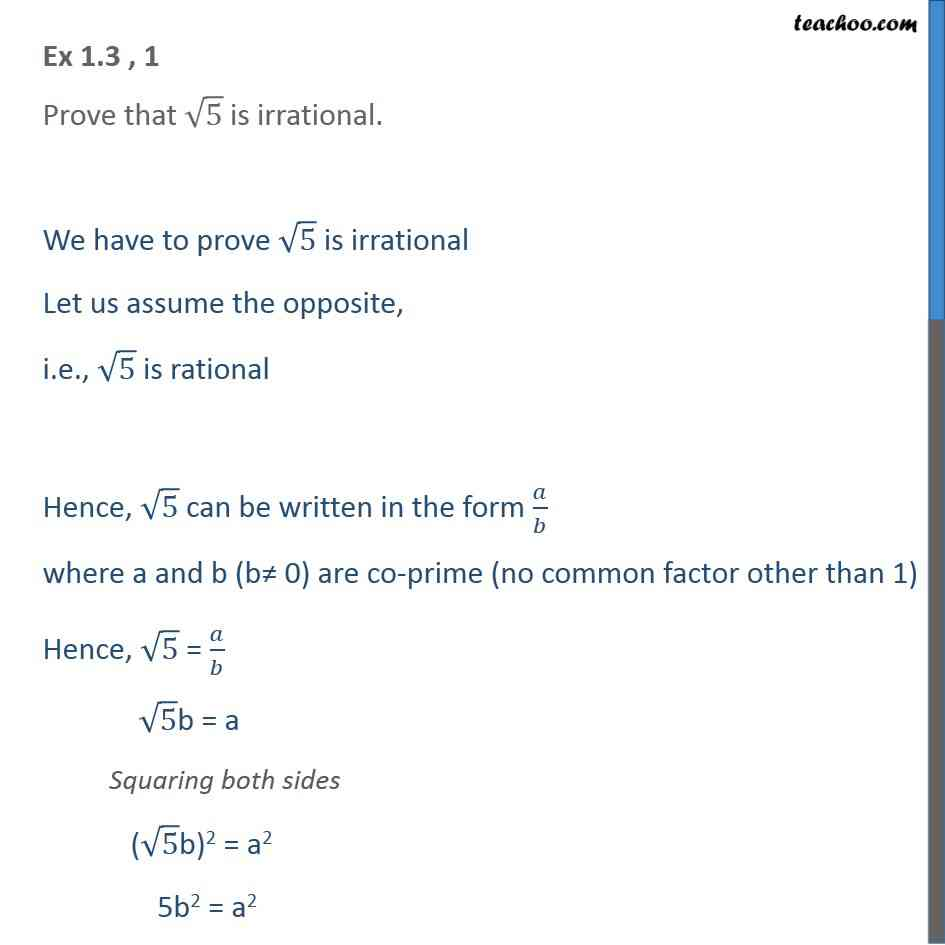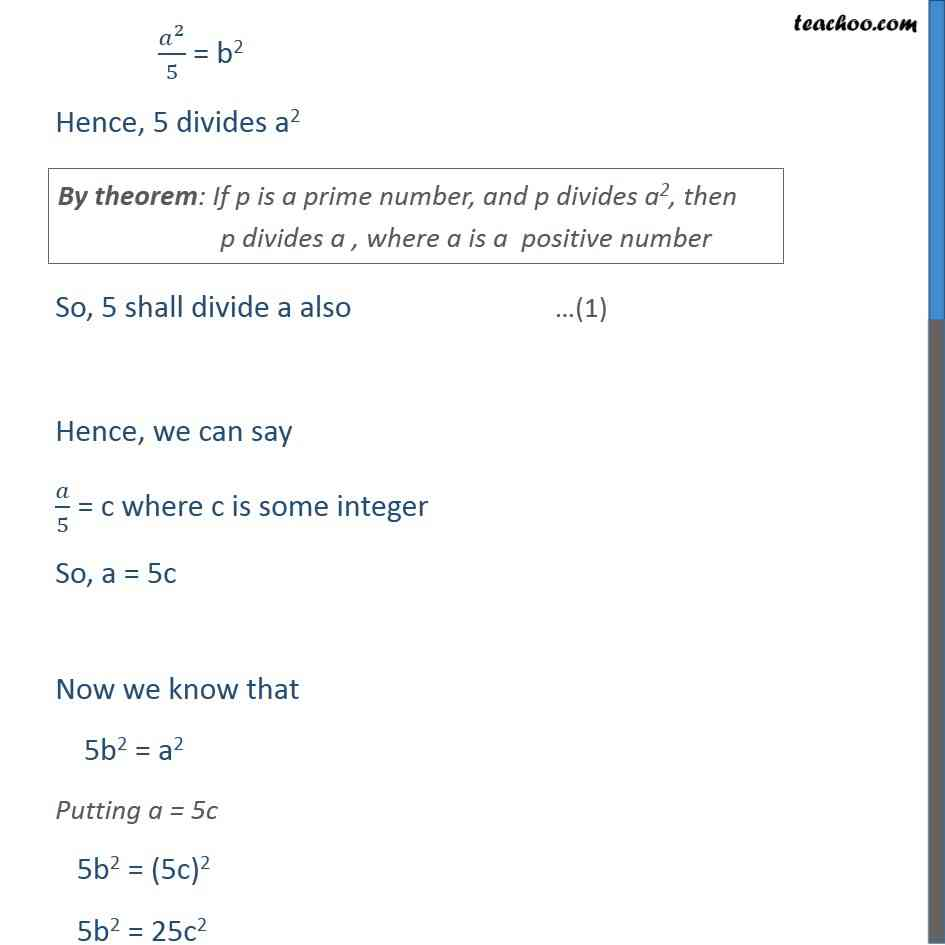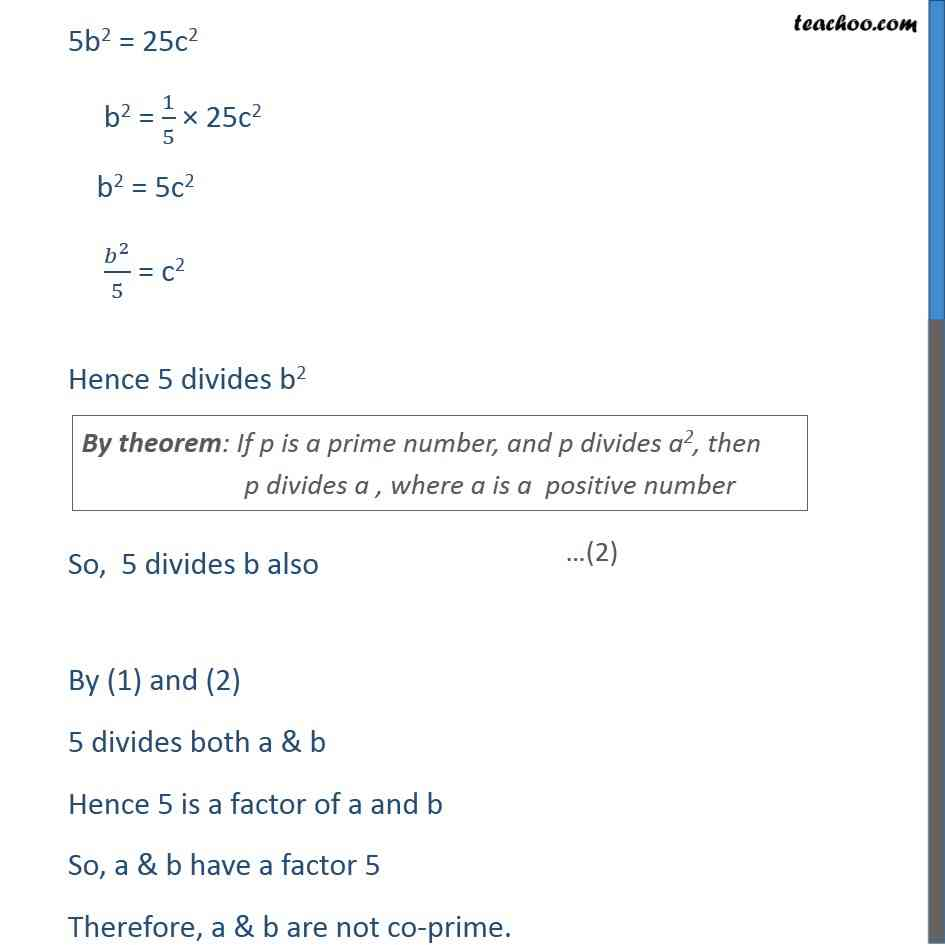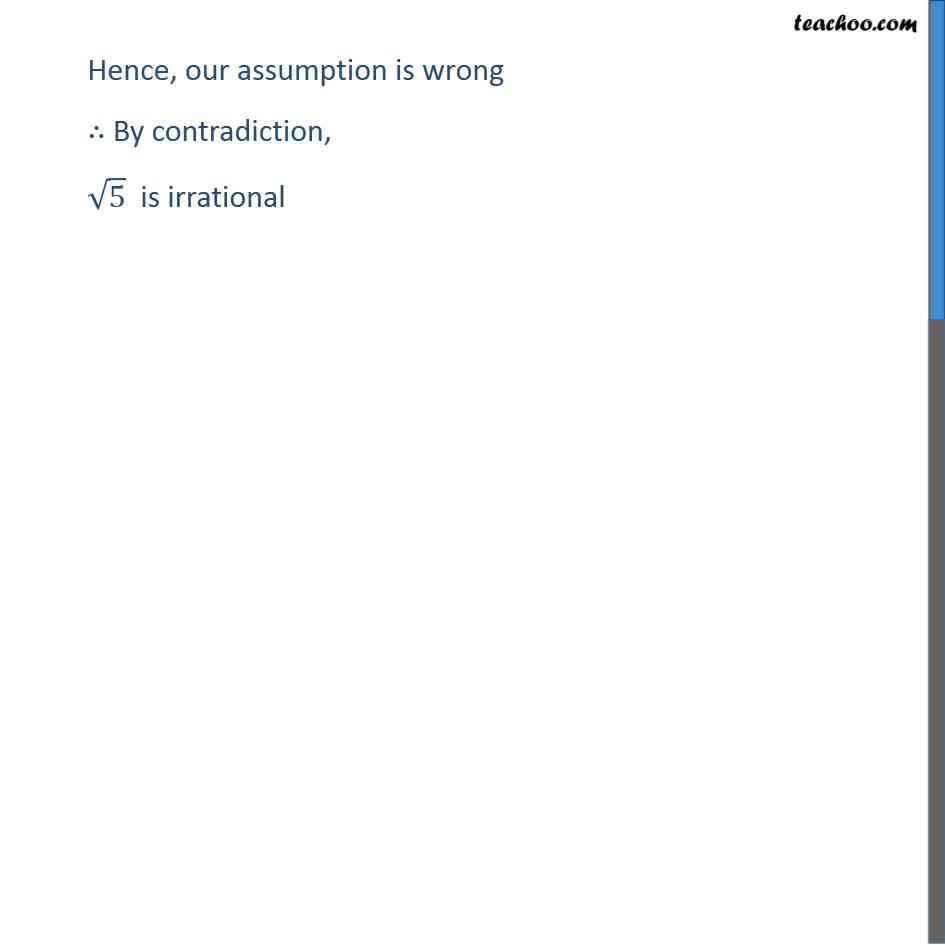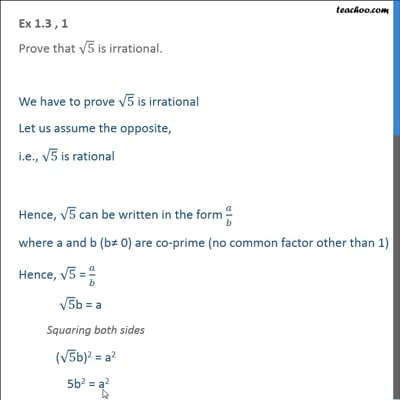This video is only available for Teachoo black users

Maths Crash Course - Live lectures + all videos + Real time Doubt solving!

### Transcript

Ex 1.3 , 1 Prove that 5 is irrational. We have to prove 5 is irrational Let us assume the opposite, i.e., 5 is rational Hence, 5 can be written in the form / where a and b (b 0) are co-prime (no common factor other than 1) Hence, 5 = / 5b = a Squaring both sides ( 5b)2 = a2 5b2 = a2 ^2/5 = b2 Hence, 5 divides a2 So, 5 shall divide a also Hence, we can say /5 = c where c is some integer So, a = 5c Now we know that 5b2 = a2 Putting a = 5c 5b2 = (5c)2 5b2 = 25c2 5b2 = 25c2 b2 = 1/5 25c2 b2 = 5c2 ^2/5 = c2 Hence 5 divides b2 So, 5 divides b also By (1) and (2) 5 divides both a & b Hence 5 is a factor of a and b So, a & b have a factor 5 Therefore, a & b are not co-prime.Hence, our assumption is wrong By contradiction, 5 is irrational